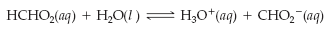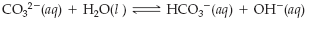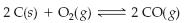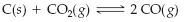×
Get Full Access to Introductory Chemistry - 5 Edition - Chapter 15 - Problem 46p
Get Full Access to Introductory Chemistry - 5 Edition - Chapter 15 - Problem 46p

×

# Solution: Write an equilibrium expression for each chemical equation involving one orISBN: 9780321910295 34

## Solution for problem 46P Chapter 15

Introductory Chemistry | 5th Edition

• Textbook Solutions
• 2901 Step-by-step solutions solved by professors and subject experts
• Get 24/7 help from StudySoup virtual teaching assistantsIntroductory Chemistry | 5th Edition

4 5 1 262 Reviews
27
2
Problem 46P

Write an equilibrium expression for each chemical equation involving one or more solid or liquid reactants or products.

(a)(b)(c)(d)Step-by-Step Solution:

Solution:

Step 1

Equilibrium expression for each chemical equations are given below:

(a)HCHO2 (aq)+  H2O (l)H3O+ (aq) + CHO2- (aq)

[H3O+] [CHO2-]

Keq   =    -------------------

[HCHO2]

Step 2 of 4

Step 3 of 4

##### ISBN: 9780321910295

This textbook survival guide was created for the textbook: Introductory Chemistry, edition: 5. Introductory Chemistry was written by and is associated to the ISBN: 9780321910295. This full solution covers the following key subjects: chemical, equation, Equilibrium, Expression, liquid. This expansive textbook survival guide covers 19 chapters, and 2046 solutions. Since the solution to 46P from 15 chapter was answered, more than 419 students have viewed the full step-by-step answer. The answer to “Write an equilibrium expression for each chemical equation involving one or more solid or liquid reactants or products.(a) (b) (c) (d)” is broken down into a number of easy to follow steps, and 21 words. The full step-by-step solution to problem: 46P from chapter: 15 was answered by , our top Chemistry solution expert on 05/06/17, 06:45PM.

Unlock Textbook Solution